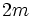# Dihedral trick

This fact is useful in work leading up to the Classification of finite simple groups

This article describes a method that can be used to prove that two elements inside a group are conjugate

## Statement

### Statement with symbols

Let$x$ and$y$ be two distinct involutions (elements of order two) in a finite group$G$. Suppose$xy$ has order$m$. Then,$\langle x, y \rangle$ is a dihedral group of order$2m$, with cyclic subgroup of order$m$ generated by$xy$ and the element$x$ of order two conjugating$xy$ to its inverse.